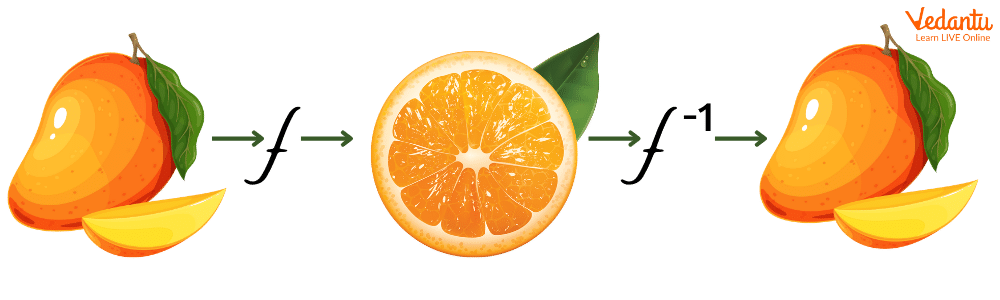Courses
Courses for Kids
Free study material
Free LIVE classes
More

# Inverse Meaning MathLIVE
Join Vedantu’s FREE Mastercalss

## Inverse Mathematics

Are you wondering to know the meaning of inverse in Mathematics? Inverse is nothing but the opposite arithmetic operation or reverse the effect of each other. For example, the inverse of addition is subtraction and the inverse of multiplication is division.

## The Inverse of Addition is Subtraction

Addition moves Forward whereas Subtraction moves Backward. Let us understand with an example:

30 + 4 = 34 can be reversed by 34 - 4 = 30. (Back to where we started)

## The Inverse of Subtraction is Addition

Let us understand inverse of subtraction with an example:

12 - 3 = 9 can be reversed by 9 + 3 = 12 (Back to where we started)

Additive inverse is what we add to the given number to get Zero.

Example:

The additive inverse of -3 is 3 because -3 + 3 = 0

Also, the additive inverse of + 8 is - 8 because 8 - 8 = 0

## The Inverse of Multiplication is Division

Multiplication can be “undone” by Division. Let us understand with an example:

Example: 6 8 = 48 can be reversed by 488 = 6 (Back to where we started)

## The Inverse of Division is Multiplication

Division can be “undone” by Multiplication. Let us understand with an example:

Example: 18 3 = 6  can be reversed by 6 3 = 18 (Back to where we started)

## Multiplicative Inverse

Multiplicative inverse is with what we multiply a given number to get the result 1.

In other words, it is simply the reciprocal of a given number. Let us understand with an example:

The multiplicative inverse of 6 is 16, because 6 16 = 1.

But Division With 0 Cannot be Done.

We cannot divide any number by 0.

Example:

6 0 = 0 cannot be reversed by 0 0.

## Inverse Function

An inverse function can be “undone” by another function.  Let us understand with an example:

Here, the function 3x + 5 is represented in a flow diagram below:

x Multiply by 3 3x Add 5 3x + 5

The inverse function goes the other way:

y - 53    Divided By 3    Y - 5   Subtract 5   y

Therefore,  the inverse of 3x+ 5 is  y - 53.

## Inverse of Function Representation

The inverse is generally represented with a little -1 after the function name as shown below:

f⁻¹ (y)

It can be said as f inverse of y

Hence, the inverse of f(x) = 3x + 5 is written as:

f⁻¹ (y) = y - 53

## Important Thing To Note About Inverse of A Function

The most important thing about the inverse of function is that it takes us back where we started.Here, the function f turns the mango into an orange.

Then, the inverse function f⁻¹ turns the orange back into the mango.

Let us understand with an example.

## Inverse Function Example

Find the inverse of 5x + 5, when the value of x is 3,

Solution:

Given function: 5x + 5

Substituting the value of x in the above function, we get

53 + 5

15 + 5 = 20

Therefore, 5x + 5 = 20 ( when the value of x is 3).

Now, we will use the inverse on 20 using the formula discussed above .

f⁻¹ (20) = 20 - 55 = 3

And now you can see, we get the 3 back again.

This can be written in one line as:

f⁻¹ (f(20)) = 3

Hence, we can say applying a function f and then its inverse f⁻¹ give us the original value back.

f⁻¹ (f(x)) = x

## Conclusion

In short, inverse means opposite in direction or position. The term ‘inverse’ is derived from the Latin word ‘inverses’ which means to turn upside down or inside and outside. In other words, an inverse operation is a mathematical operation that undoes what was performed on the previous operation.

Last updated date: 01st Oct 2023
Total views: 141.3k
Views today: 4.41k

## FAQs on Inverse Meaning Math

1. What is the inverse function in Mathematics?

Inverse function is a Mathematical function that undoes the effect of another function. For example, the inverse function formula to convert Celsius to Fahrenheit temperature is the same formula we use to convert Fahrenheit temperature  to Celsius temperature.

2. What is the difference between inverse and reciprocal?

Inverse and reciprocal both are widely used in Mathematics and have the same meanings. The reciprocal or multiplicative inverse of ‘x’ is represented as 1x. This implies, if we have a fraction pq, its multiplicative inverse or reciprocal will be qp.  Also, if you have a real number 72, just divide 72 by 1 to get its inverse or reciprocal. Therefore, the inverse of 72  will be 27 ( 1 72  = 1 27  = 27 ).